## Business Weekly conducted a survey of graduates from 30 top MBA programs. On the basis of the survey, assume the mean annual salary for grad

Question

Business Weekly conducted a survey of graduates from 30 top MBA programs. On the basis of the survey, assume the mean annual salary for graduates 10 years after graduation is 181000 dollars. Assume the standard deviation is 31000 dollars. Suppose you take a simple random sample of 60 graduates.

Find the probability that a single randomly selected policy has a mean value between 172595.6 and 196608.1 dollars.
P(172595.6 < X < 196608.1) =

Find the probability that a random sample of size
n
=
60
has a mean value between 172595.6 and 196608.1 dollars.
P(172595.6 < M < 196608.1) =

in progress 0
5 months 2021-09-01T10:48:50+00:00 1 Answers 3 views 0

0.2979 = 29.79  probability that a single randomly selected policy has a mean value between 172595.6 and 196608.1 dollars.

0.982 = 98.2% probability that a random sample of size 60 has a mean value between 172595.6 and 196608.1 dollars.

Step-by-step explanation:

To solve this question, we need to understand the normal probability distribution and the central limit theorem.

Normal Probability Distribution:

Problems of normal distributions can be solved using the z-score formula.

In a set with mean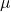and standard deviation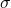, the z-score of a measure X is given by: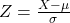The Z-score measures how many standard deviations the measure is from the mean. After finding the Z-score, we look at the z-score table and find the p-value associated with this z-score. This p-value is the probability that the value of the measure is smaller than X, that is, the percentile of X. Subtracting 1 by the p-value, we get the probability that the value of the measure is greater than X.

Central Limit Theorem

The Central Limit Theorem establishes that, for a normally distributed random variable X, with meanand standard deviation, the sampling distribution of the sample means with size n can be approximated to a normal distribution with meanand standard deviation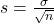.

For a skewed variable, the Central Limit Theorem can also be applied, as long as n is at least 30.

On the basis of the survey, assume the mean annual salary for graduates 10 years after graduation is 181000 dollars. Assume the standard deviation is 31000 dollars.

This means that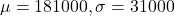Find the probability that a single randomly selected policy has a mean value between 172595.6 and 196608.1 dollars.

This is the p-value of Z when X = 196608.1 subtracted by the p-value of Z when X = 172595.6. So

X = 196608.1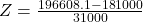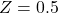has a p-value of 0.6915

X = 172595.6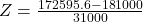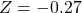has a p-value of 0.3936

0.6915 – 0.3936 = 0.2979

0.2979 = 29.79  probability that a single randomly selected policy has a mean value between 172595.6 and 196608.1 dollars.

Sample of 60:

This means that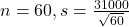Now, the probability is given by:

X = 196608.1By the Central Limit Theorem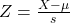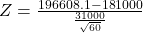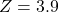has a p-value of 0.9999

X = 172595.6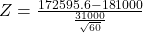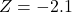has a p-value of 0.0179

0.9999 – 0.0179 = 0.982

0.982 = 98.2% probability that a random sample of size 60 has a mean value between 172595.6 and 196608.1 dollars.